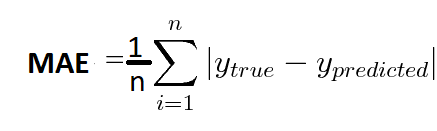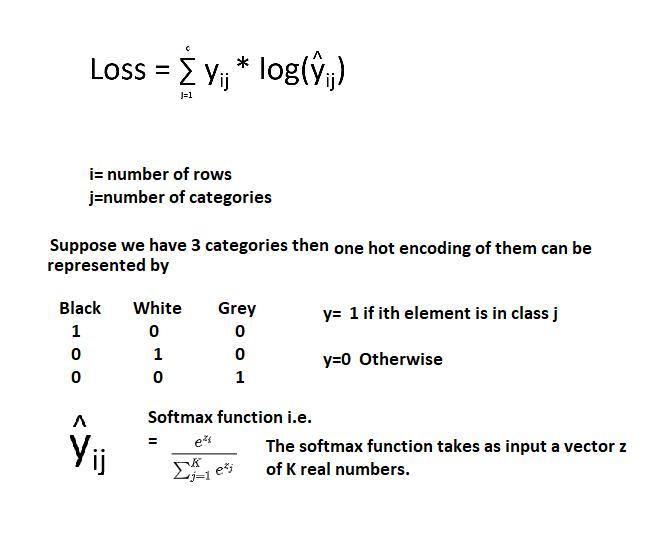Search

# Loss Functions -when to use which one

The ultimate goal of all algorithms of machine learning is to decrease loss. Loss has to be calculated before we try strategy to decrease it using different optimizers.

Loss function is sometimes also referred as Cost function.

Loss Function is an error in 1 data point while Cost Error Function is sum of all errors in a batch of dataset.

There are two types of models in machine learning, regression and classification, the loss functions of both are different. Lets discuss first about Regression problem losses first

Cost functions in Regression Problems

1) Mean Squared Error

MSE measures the average of the sum of squares of the errors. It averages squared difference between the estimated values and the actual value. It is a kind of risk function where it calculates the deviation from the actual value with the predicted value which is squared and averaged with the number of instances a model has.

MSE is almost always positive is because of randomness or because the estimator does not calculate a negative value as it is squared.

The MSE values closer to zero are better as this refers that model has less error.

The function formula is as below:n here is batch size.

a) In the form of quadratic equation, when we plot a quadratic equation, we get a gradient descent with only one global minima.

b) There is no local minima.

c) It penalizes the model for making larger errors by squaring them. Example y-y^ is big then it will become bigger if it is squared.

a) Outliers are not handled properly. As outlier error will be quite large, it is penalized squaring it.

2) Mean Absolute Error

It can be called as arithmetic average of absolute errors, i.e. absolute difference between actual and predicted paired data points. The formula is written below:n here is batch size.

a) Outliers are handled better than MSE as it is not penalizing the model by squaring error value.

a) It is computationally expensive as it uses modulus operator function.

b) There may be a local minima.

3) Huber Loss

Huber Loss is often used in regression problems. Compared with MSE, Huber Loss is less sensitive to outliers as if the loss is too much it changes quadratic equation to linear and hence is a combination of both MSE and MAE.a) Outliers are handled properly.

b) Local minima situation is handled here.

a) In order to maximize model accuracy, the hyperparameter δ will also need to be optimized which increases the training requirements.

b) It is complex.

Classification Problems Loss functions

Cross Entropy Loss

1) Binary Cross Entropy-Logistic regression

If you are training a binary classifier, then you may be using binary cross-entropy as your loss function.

Entropy as we know means impurity. The measure of impurity in a class is called entropy. SO loss here is defined as the number of the data which are misclassified.

We know that in binary classification problem Sigmoid function is used to calculate the output. Sigmoid which is used in logistic regression model for classification.

The formula for it is as under.

Since log is used here we will see as the entropy as the probability of getting a true class decreases or nears zero the loss increases.2) Multi-Class Cross Entropy

For Multiclass problems mostly Softmax function is used to classify the dataset. For classification the data is subjected to one-hot encoding technique.Sigmoid-cross-entropy-loss uses sigmoid to convert the score vector into a probability vector, and softmax cross entropy loss uses a softmax function to convert the score vector into a probability vector.

These are high level Loss functions that can be used in regression and classification problems. Hope it clarifies the major loss functions.